Return to Contents

# Solutions to the Critical Section Problem

Assumption

• assume that a variable (memory location) can only have one value; never ``between values''
• if processes A and B write a value to the same memory location at the ``same time,'' either the value from A or the value from B will be written rather than some scrambling of bits

Peterson's Algorithm

• a simple algorithm that can be run by two processes to ensure mutual exclusion for one resource (say one variable or data structure)
• does not require any special hardware
• it uses busy waiting (a spinlock)

Peterson's Algorithm
Shared variables are created and initialized before either process starts. The shared variables flag and flag are initialized to FALSE because neither process is yet interested in the critical section. The shared variable turn is set to either 0 or 1 randomly (or it can always be set to say 0).

var flag: array [0..1] of boolean;
turn: 0..1;

%flag[k] means that process[k] is interested in the critical section
flag := FALSE;
flag := FALSE;
turn := random(0..1)

After initialization, each process, which is called process i in the code (the other process is process j), runs the following code:

repeat
flag[i] := TRUE;
turn := j;
while (flag[j] and turn=j) do no-op;
CRITICAL SECTION
flag[i] := FALSE;
REMAINDER SECTION
until FALSE;

Information common to both processes:
turn = 0
flag = FALSE
flag = FALSE

 EXAMPLE 1 Process 0 Process 1 i = 0, j = 1 i = 1, j = 0 flag := TRUE turn := 1 check (flag = TRUE and turn = 1) -  Condition is false because flag = FALSE - Since condition is false, no waiting in while loop -  Enter the critical section -  Process 0 happens to lose the processor flag := TRUE turn := 0 check (flag = TRUE and turn = 0) - Since condition is true, it keeps busy waiting until it loses the processor - Process 0 resumes and continues until it finishes in the critical section - Leave critical section flag := FALSE - Start executing the remainder (anything else a process does besides using the critical section) - Process 0 happens to lose the processor check (flag = TRUE and turn = 0) - This condition fails because flag = FALSE - No more busy waiting - Enter the critical section

 EXAMPLE 2 Process 0 Process 1 i=0, j=1 i=1, j=0 flag = TRUE turn = 1 - Lose processor here flag := TRUE turn := 0 check (flag = TRUE and turn = 0) - Condition is true so Process 1 busy waits until it loses the processor check (flag = TRUE and turn = 1) -  This condition is false because turn = 0 - No waiting in loop - Enters critical section

 EXAMPLE 3 Process 0 Process 1 i=0, j=1 i=1, j=0 flag = TRUE - Lose processor here flag = TRUE turn = 0 check (flag = TRUE and turn = 0) - Condition is true so, Process 1 busy waits until it loses the processor turn := 1 check (flag = TRUE and turn = 1) - Condition is true so Process 0 busy waits until it loses the processor check (flag = TRUE and turn = 0) - The condition is false so, Process 1 enters the critical section

Summary of Techniques for Critical Section Problem
Software

1. Peterson's Algorithm: based on busy waiting
2. Semaphores: general facility provided by operating system (e.g., OS/2)
• based on low-level techniques such as busy waiting or hardware assistance
• described in more detail below
3. Monitors: programming language technique
• see S&G, pp. 190--197 for more details

Hardware

• always assumed
2. Interrupts that can be turned off
• must have only one processor for mutual exclusion
3. Test-and-Set: special machine-level instruction
• described in more detail below
4. Swap: atomically swaps contents of two words
• see S&G, pp. 173--174 for more details
• for example, the Exchange instruction is available in the machine language used on Intel processors.

Test-and-Set

• hardware assistance for process synchronization
• a special hardware instruction that does two operations atomically
• i.e., both operations are executed or neither is
• sets the result to current value
• changes current value to true
• when describing machine language (CPU) operations, the verb 'set' means 'set to true'

Test-and-Set Solution to the Critical Section Problem

repeat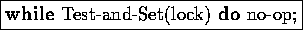critical section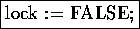remainder section

until false

Test-and-Set(target)

result := target
target := TRUE
return result

Information common to both processes:
target = FALSE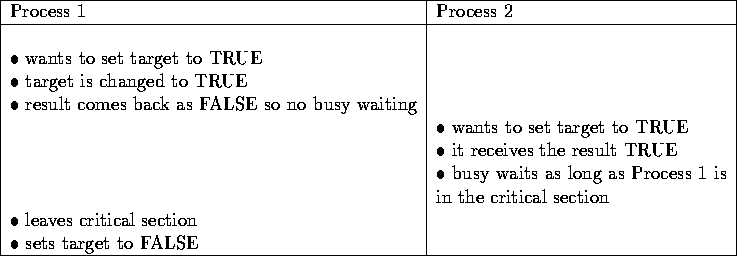Swap

• another type of hardware assistance for process synchronization
• a special hardware instruction that does a complete swap (ordinarily three operations) atomically
• i.e., the complete swap is executed or no part of it is
• given pass-by-reference parameters A and B, it swaps their values

Swap Solution to the Critical Section Problem

• uses two variables called lock and key
• intuition: if lock is false, then a process can enter the critical section, and otherwise it can't
• initially, lock is false

repeat

var = true
while (var == true) swap(lock, var);
critical section
lock = false;
remainder section

until false

Semaphores

• originally, semaphores were flags for signalling between ships
• a variable used for signalling between processes

·         operations possible on a semaphore:

o        initialization

• done before individual processes try to operate on the semaphore

o        two main operations:

• wait (or acquire)
• signal (or release)

o        the wait and signal operations are atomic operations (e.g., the test-and-set at the top of the loop of wait is done before losing the processor)

o        e.g., a resource such as a shared data structure is protected by a semaphore. You must acquire the semaphore before using the resource and release the semaphore when you are done with the shared resource.

wait(S):

while S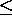0 do

no-op;

S.value := S.value - 1;

signal(S):

S := S + 1;

In either case, the initial value for S:

1. equals 1 if only one process is allowed in the critical section (binary semaphore)
2. equals n if at most n processes are allowed in the critical section

Semaphore Solution to the Critical Selection Problem
repeat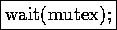critical section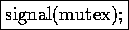remainder section
until false;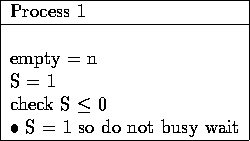Alternative Implementation of Wait and Signal

wait(S): this code is executed atomically

S.value := S.value - 1;
if S.value < 0
then begin

suspend this process;

end;actual waiting is done by the suspended process

signal(S):

S.value := S.value + 1;
if S.value0
then begin

remove a process P from S.L;
wakeup(P);

end;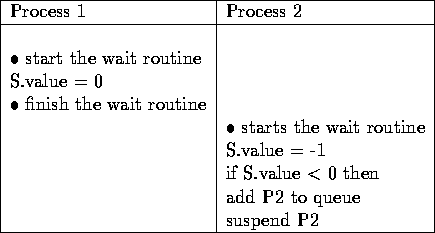mutex: a binary semaphore used to enforce mutual exclusion (i.e., solve the critical section problem)

Implementing Semaphores
To ensure the atomic property, the OS implementation must either:

• turn off interrupts
• use busy waiting: to make sure only one process does a wait operation at once
• Peterson's Algorithm
• Test-and-Set
• Swap

Bounded Buffer Problem (Producer/Consumer Problem)for example, in UNIX a pipe between two processes is implemented as a 4Kb buffer between the two processes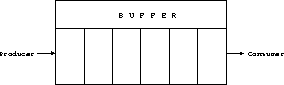Producer

• creates data and adds to the buffer
• do not want to overflow the buffer

Consumer

• removes data from buffer (consumes it)
• does not want to get ahead of producer

Information common to both processes:
empty := n
full := 0
mutex := 1
Producer Process

repeat

produce an item in nextp

wait(empty);
wait(mutex);

signal(mutex);
signal(full);

until false;

Consumer Process
repeat

wait(full);
wait(mutex);

remove an item from buffer to nextc

signal(mutex);
signal(empty);

consume the item in nextc

until false;

mutex: (semaphore) initialized to 1
empty: count of empty locations in the bufferinitialized to n, the buffer size
full: count of full locations in the bufferinitialized to 0The empty and full semaphores are used for process synchronization. The mutex semaphore is used to ensure mutually exclusive access to the buffer.
if we were to change the code in the consumer process from:
wait(full)
wait(mutex)

to
wait(mutex)
wait(full)
,
then we could reach a deadlock where Process 1 is waiting for Process 2 and Process 2 is waiting for Process 1.Return to Contents